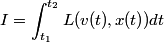## Solution to 1986 Problem 36

 The Lagrangian of the system is\begin{align*}L = \frac{1}{2}m v^2 - k x^4\end{align*}According to Hamilton's principle, a particle will along the trajectory that minimizes the action\begin{align*}I = \int_{t_1}^{t_2} L(v(t),x(t)) dt\end{align*}Therefore, answer (A) is correct.